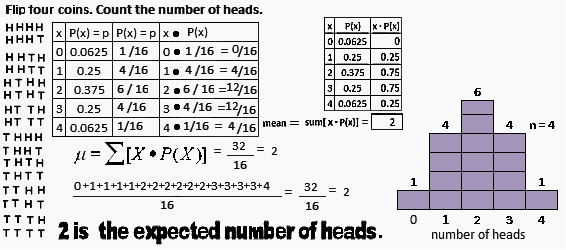### MIDDLE GROUND - Some Features of A Binomial Distribution

I.   Brief Summary of A Binomial Distribution
0.   Basic Probability and Counting Formulas
Vocabulary, Facts, Count the Ways to Make An Ordered List Or A Group
The average is the sum of the products of the event and the probability of the event.
II.   Binomial Distribution Explained More Slowly
III. Binomial Formula Explained
Combinations Compute The Number of Each Outcome in A Binomial Distribution
What's the Probability of Obtaining Exactly 3 Heads If A Fair Coin Is Tossed 4 Times?
Applications
IV. Sum of the Probabilities and the Binomial Mean
The Sum of The Probabilities Is One.
The Expected Value Is The Mean.
The Mean, Expected Value, Is (n)(p).
Why the mean, expected value, is (n)(p)
V.   Examples
VI. Use the Normal to Compute the Binomial on a Calculator

 The Sum of The Probabilities Is One.         This page continues to illustrate probability facts using the flip-a-coin-4-times-and-count-the-number-of-heads problem. These facts are mentioned on the Basic Probability page and the Breif Summary of the Binomial Distribution page.The lowest possible probability an outcome might have is 0. P(x=7)=0, the probability of getting 7 heads, when only 4 coins are flipped, is 0. The event is not possible.  The highest possible probability an outcome might have is 1. P(x=0 or x=1 or x=2 or x=3 or x=4)=1. The event does happen.  Probabilities range between 0 and 1, inclusive. p(x=0)=1/16, p(x=1)=4/16, p(x=2)=6/16, p(x=3)=4/16, p(x=4)=1/16. Each of these is between 0 and 1, inclusive.  The sum of all the probabilities for an experiment is 1. p(x=0) + p(x=1) + p(x=2) + p(x=3) + p(x=4) = 16/16 = 1  Below other binomial distributions are summarized.When 1 coin is tossed,     p(x=0) + p(x=1) = 1/2 + 1/2 = 1  When 2 coins are tossed,     p(x=0) + p(x=1) + p(x=2) = 1/4 + 2/4 + 1/4 = 1  When 3 coins are tossed,     p(x=0) + p(x=1) + p(x=2) + p(x=3) = 1/8 + 3/8 + 3/8 + 1/8 = 1  When 5 coins are tossed,     p(x=0) + p(x=1) + p(x=2) + p(x=3) + p(x=4)+ p(x=5)=       1/32 + 5/32 + 10/32 + 10/32 + 5/32 + 1/32 = 32/32 = 1

 The Mean is the Expected Value         A mean is an average or center of a set of numbers or data. The mean is also, by definition and in the same sense it would have in English, the expected value.The expected value is the mean. Toss 1 coin,= (0+1)/2 = 1/2 Toss 2 coins,= (0+1+1+2)/4 = 4/4 = 1 Toss 3 coins,= (0+1+1+1+2+2+2+3)/8 = 12/8 = 1.5 Toss 4 coins,= (0+1+1+1+1+2+2+2+2+2+2+3+3+3+3+4)/16 = 32/16 = 2 Toss 5 coins,= (0+1+1+1+1+1+2+2+2+2+2+2+2+2+2+2+3+3+3+3+3+3+3+3+3+3+4+4+4+4+4+5)/32 = 80/32 = 2.5

 The Mean, Expected Value, Is (n)(p).         To compute the mean, expected value, find the sum of the products of event and probability of event.         The expected value is the mean. [See a more detailed reason.]For a binomial distribution, to compute the mean, expected value, multiply the number of trial by the probability of success on a trial.The expected value is the mean, (n)(p). Here a fair coin is used so p = 1/2, or .5 and n varies.  Toss 1 coin, n=1 so= n(p) = 1(1/2) = 1/2 =.5 Toss 2 coins, n=2 so= n(p) = 2(1/2) = 1 Toss 3 coins, n=3 so= n(p) = 3(1/2) = 3/2 = 1.5 Toss 4 coins, n=4 so= n(p) = 4(1/2) = 2 Toss 5 coins, n=5 so= n(p) = 5(1/2) = 5/2 = 2.5© 2010, Agnes Azzolino www.mathnstuff.com/math/spoken/here/2class/90/binom4.htm